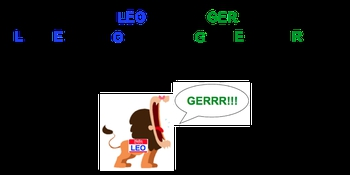Start typing, then use the up and down arrows to select an option from the list.## General Chemistry

Learn the toughest concepts covered in Chemistry with step-by-step video tutorials and practice problems by world-class tutors

6. Chemical Quantities & Aqueous Reactions

Redox Reactions (oxidation-reduction reactions) involve transference of an electron(s) between reactants.

Oxidation-Reduction Reactions
1
concept

## Redox Reactions1m
Play a video:
redox reactions, also known as oxidation reduction reactions involved transference of an electron or electrons between reactors. And now a memory tool that will help us remember. What does it mean to be oxidized versus reduced? Is that leo the lion goes ger So leo stands for lose electrons. Oxidation anger stands for gain electrons reduction. So let's go back and think about this, lose electrons. Oxidation electrons are negatively charged. If I'm losing something that's negative, then my life me as a substance becomes more positive, so losing the negative to become more positive. So you're becoming more positive as you lose negative electrons. But let's talk about this in terms of oxidation numbers, I'm becoming more positive. That means my oxidation number is increasing. Maybe I go from plus two, two plus four. Now, if you're gaining negative things in your life, which we all don't want to happen, you're gonna become more negative. So you become more negative in charge. What does that mean in terms of oxidation number? Well, if you are becoming more negative, that means that your number is decreasing. So your oxidation number is going down. So maybe I go from plus three to minus one, my oxidation number has decreased. So just remember leo means lose electrons, oxidation, I become more positive. My oxidation number goes up, gain electrons, reduction, I become more negative. My oxidation number decreases. We're gonna remember this when we take a look at redox reactions

To remember the difference between oxidation and reduction just learn:LEO the lion goes GER2
concept

## Redox Reactions1m
Play a video:
now usually accompanied with the terms off reduction and oxidation are the ideas of the reducing agent and the oxidizing agent. But what you need to realize here is that reduction and oxidation are the opposites off oxidizing and reducing. Agents were going to say the oxidizing agent is just the element or compound that is reduced and then reducing Agent is the element or compound that is oxidized. So add this to your idea of Leo the Lion goes girl. Okay, so if you've been oxidized, you're the reducing agent. If you've been reduced, your the oxidizing agent, I know it could be a little bit weird, but just remember, they're opposites of one another. If we can remember that, they will be able to solve Redox reaction questions that ask us to identify what's been oxidized, what's been reduced. What's the oxidizing agent and what's the reducing agent

Oxidizing Agent is reduced and in turn oxidizes another element/compound.

Reducing Agent is oxidized and in turn reduces another element/compound.

3
example

## Redox Reactions Example 12m
Play a video:
in this example question, it says. Consider the following reaction below when solid lithium reacts with the zinc ion. So here we have two moles of lithium solid reacting with one mole of zinc ion in its acquiesce state, and it produces two moles of lithium ion in its acquiesced eight plus zinc solid. The question says, which reactant is undergoing oxidation and which reacting is undergoing reduction. So if we take a look here, lithium solid is in its natural state, So lithium solid oxidation number initially is zero. Zinc here is in its ionic form. It has its charge present. Remember, if we have the charge of the ion, then that can equal the oxidation number. So here this will be plus two going to the other side. We have lithium ion again. It's in its ionic form. If the charge is present, then that charges connected to the oxidation number, so it's plus one and then zinc is now in its natural state, so it's zero. So when we're talking about what's been oxidized, what's been reduced, we look at the reactions. So we have lithium solid and we have what zinc two plus lithium as a reactant goes from having an oxidation number of zero to an oxidation number of plus one. We're gonna say that it's oxidation. Number went up, It increased. It went from zero to plus one. Remember, if you're oxidation number increases, that means that you've been oxidized, So lithium solid has been oxidized. Zinc zinc starts off with plus two as it's oxidation number. And then as a product, it goes down to zero. We see that it's oxidation number decreased. Remember, if you're oxidation number decreased, you've been reduced. So zinc two plus ion has been reduced. Remember what's been oxidized and reduced is the reacted. We look on the product side just to see how our oxidation number has changed. But again, it is the reactant that are oxidized or reduced. In this case, it would give us lithium solid as being oxidized and zinc two plus ion as being reduced
4
Problem

Which element is being reduced in the following reaction?

Cr2O72- + 3 HNO2 + 5 H→ 2 Cr3+ +  3NO3- + 4 H2O

5
Problem

Identify the oxidizing agent and reducing agent from the following redox reaction.

Ba (s) + Cl2 (g) → BaCl2 (aq)

6
Problem

Which element is oxidized and which is reduced in the following reaction?

Hg (aq) + HgCl2 (aq) → Hg2Cl2

7
Problem

Which of the following represents an oxidation-reduction reaction?

I. PCl3 (aq) + Cl2 (g) → PCl5 (aq)

II. 2 AgNO3 (aq) + Cu (s) → Cu(NO3)2 (aq) + 2 Ag (s)

III. CO2 (g) + 2 LiOH (aq) → Li2CO3 (aq) + H2O (l)

IV. FeCl2 (aq) + 2 NaOH (aq) → Fe(OH)2 (aq) + 2 NaCl (aq)